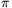Mathematical and Physical Journal
for High Schools
Issued by the MATFUND Foundation
 Already signed up? New to KöMaL?

#Problem A. 556. (February 2012)

A. 556. Prove that for arbitrary real numbersthere exist a real t such that(5 pont)

Deadline expired on March 12, 2012.

Solution. Define the functionand let. We will show that. Then it follows that at least one of the choices t=a1, ..., t=an proves the statement.

The role of a1,...,an is symmetric and the function |sin x| is periodic by, so without loss of generality we may assume. Define a0=0 too; then f(a0)=f(an).

If, then, and the statement is trivial. In the rest of the solution we assume a1<as well; then.

By the periodicity of |sin x|,(1)

Now we prove that(2)

for all 1kn.

We prove (2) termwise. For each index 1in,In the interval [ak-1,ak] the function sin (x-ai) has constant sign: it is nonnegative for ik-1, and nonpositive for ik. Multiplying by (-1) for i<k and summing up we obtain (2).

Combining (1) and (2), and applying Jensen's inequality to the tangent function (which is convex in [0,/2), we get### Statistics:

 6 students sent a solution. 5 points: Gyarmati Máté, Janzer Olivér, Omer Cerrahoglu. 4 points: Machó Bónis. 0 point: 2 students.

Problems in Mathematics of KöMaL, February 2012